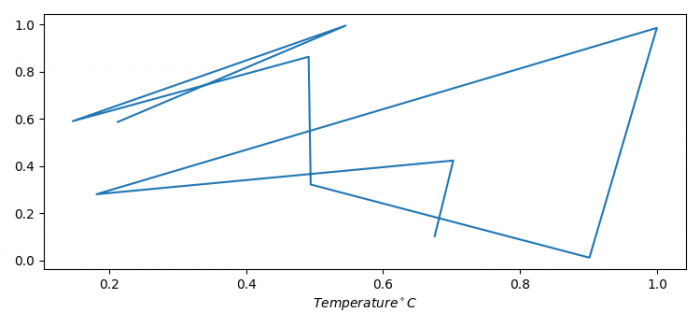# How do I print a Celsius symbol with Matplotlib?

To print Celsius symbol with Matplotlib, we can take the following steps −

• Set the figure size and adjust the padding between and around the subplots.
• Initialize a variable, N.
• Create T and P data points using numpy.
• Plot T and P using plot() method.
• Set the label for the X-axis.
• To display the figure, use show() method.

## Example

import matplotlib.pyplot as plt
import numpy as np

plt.rcParams["figure.figsize"] = [7.50, 3.50]
plt.rcParams["figure.autolayout"] = True

N = 10
T = np.random.rand(N)
P = np.random.rand(N)

plt.plot(T, P)
plt.xlabel("$Temperature {^\circ}C$")

plt.show()

## Output